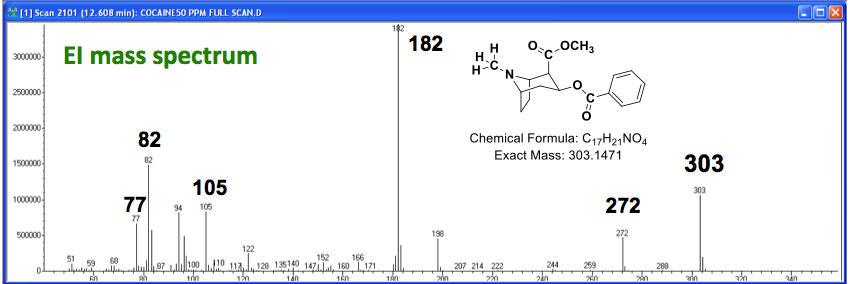# Mass Spectrometry: Nitrogen Rule

## Exercise 1.

This exercise will walk you through the basics of the nitrogen rule as well its application to the EI and ESI mass spectra of cocaine.

The nitrogen rule states that any molecule (with all paired electrons) that contains an odd number of nitrogen atoms will have an odd nominal mass. The nominal mass is the integer mass of an atom, ion, or molecule comprised of only the most stable isotope(s).

1. Demonstrate that the nitrogen rule really works by calculating the nominal mass of two molecules of your choosing, one with an even number of (or zero) nitrogen atoms, the other with an odd number of nitrogen atoms.

2. Fill in the following table and describe how nitrogen is unique.

Atom

Nominal Mass

Number of Valence Electrons

Carbon

4

Hydrogen

16

Nitrogen

19

1. If a molecule (with all paired electrons) contains one nitrogen atom, why is an odd nominal mass inevitable?

Refer to the EI and ESI mass spectra of cocaine below.  Fragmentation patterns for EI (which produces OE+. molecular ions) often follow the following pattern:  OE+. → EE+ → EE+ → EE+

[Note: OE+. and EE+ correspond to an odd electron and an even electron ion, respectively.]

[Note: ESI always produces even-electron ions EE+ (if in positive mode) or EE (if in negative mode).]

1. For the following labeled ions in each spectrum, identify whether it is has an even or odd number of electrons, whether it is an even or odd nominal mass ion, and how many nitrogen atoms it has.EI:

Ion Even or Odd Electron? Even or Odd Mass? Number of Nitrogens?
m/z 77
m/z 82
m/z 105
m/z 182
m/z 272
m/z 303ESI:

Ion Even or Odd Electron? Even or Odd Mass? Number of Nitrogens?
m/z 304.155

## Exercise 2.

Consider the electron ionization (EI) mass spectrum below for methyl undecanoate, a fatty acid methyl ester.  No nitrogens are present in the molecule.  Fill in the table below. Are the fragment ions odd or even electron ions? Propose a likely structure for the fragments at m/z 74 and 169. Why is the fragment at m/z 74 is characteristic of methyl esters?

Ion

Odd or Even Mass?

Odd or Even Electron?

m/z 200

m/z 169

m/z 87

m/z 74